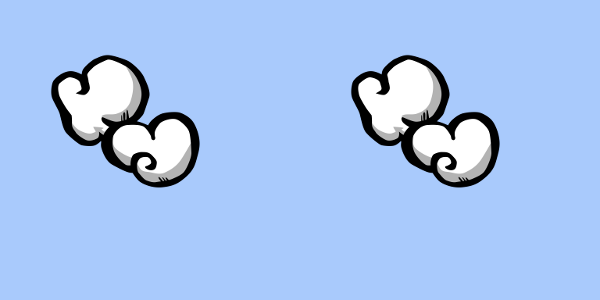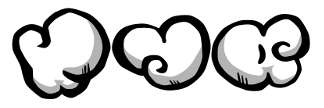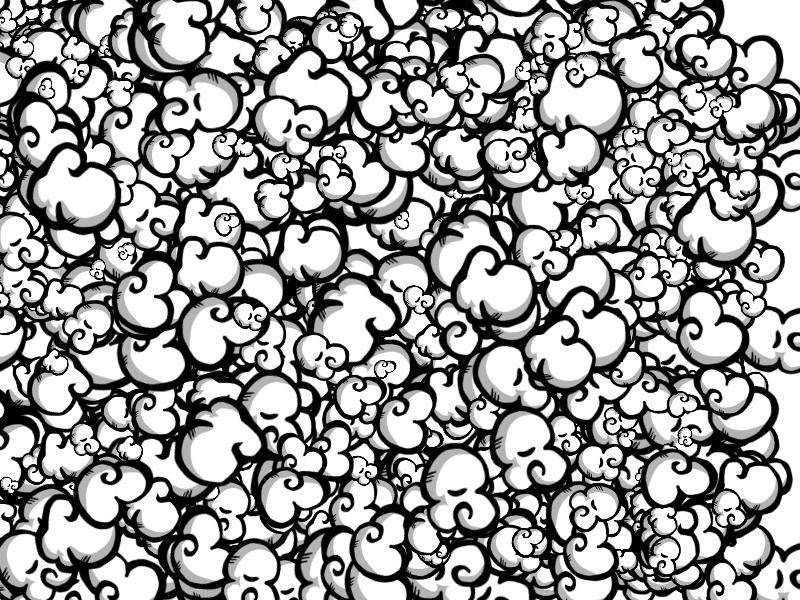# Drawing images#

## From an Image widget#

You can draw from an Image widget directly, this is the less optimized solution but it works perfectly fine if you don’t draw more than a hundred images at a time.

• `draw_image(image, x=0, y=0, width=None, height=None)`:

Draw an `image` on the Canvas at the coordinates (`x`, `y`) and scale it to (`width`, `height`). The `image` must be an `Image` widget or another `Canvas`. If `width`/`height` is `None`, the natural image `width`/`height` is used.

```from ipywidgets import Image

from ipycanvas import Canvas

sprite1 = Image.from_file("sprites/smoke_texture0.png")
sprite2 = Image.from_file("sprites/smoke_texture1.png")

canvas = Canvas(width=300, height=300)

canvas.fill_style = "#a9cafc"
canvas.fill_rect(0, 0, 300, 300)

canvas.draw_image(sprite1, 50, 50)
canvas.draw_image(sprite2, 100, 100)

canvas
```## From another Canvas#

You can draw from another `Canvas` widget. This is the fastest way of drawing an image on the canvas.

```canvas2 = Canvas(width=600, height=300)

# Here ``canvas`` is the canvas from the previous example
canvas2.draw_image(canvas, 0, 0)
canvas2.draw_image(canvas, 300, 0)

canvas2
```## From a NumPy array#

You can directly draw a NumPy array of pixels on the `Canvas`, it must be a 3-D array of integers and the last dimension must be 3 or 4 (rgb or rgba), with values going from `0` to `255`.

• `put_image_data(image_data, x=0, y=0)`:

Draw an image on the Canvas. `image_data` should be a NumPy array containing the image to draw and `x` and `y` the pixel position where to draw (top left pixel of the image).

```import numpy as np

from ipycanvas import Canvas

x = np.linspace(-1, 1, 600)
y = np.linspace(-1, 1, 600)

x_grid, y_grid = np.meshgrid(x, y)

blue_channel = np.array(np.sin(x_grid**2 + y_grid**2) * 255, dtype=np.int32)
red_channel = np.zeros_like(blue_channel) + 200
green_channel = np.zeros_like(blue_channel) + 50

image_data = np.stack((red_channel, blue_channel, green_channel), axis=2)

canvas = Canvas(width=image_data.shape, height=image_data.shape)
canvas.put_image_data(image_data, 0, 0)

canvas
```## Optimizing drawings#

Drawing from another `Canvas` is by far the fastest of the three solutions presented here. So if you want to draw the same image a thousand times, it is recommended to first draw this image on a temporary canvas, then draw from the temporary canvas a thousand times.

```from random import choice, randint, uniform
from math import pi

from ipywidgets import Image, HBox

from ipycanvas import Canvas, hold_canvas

# Create temporary Canvases
canvas_sprite1 = Canvas(width=100, height=100)
canvas_sprite1.draw_image(Image.from_file("sprites/smoke_texture0.png"), 0, 0)

canvas_sprite2 = Canvas(width=100, height=100)
canvas_sprite2.draw_image(Image.from_file("sprites/smoke_texture1.png"), 0, 0)

canvas_sprite3 = Canvas(width=100, height=100)
canvas_sprite3.draw_image(Image.from_file("sprites/smoke_texture2.png"), 0, 0)

sprites = [canvas_sprite1, canvas_sprite2, canvas_sprite3]

# Display them horizontally
HBox(sprites)
``````canvas = Canvas(width=800, height=600)

with hold_canvas():
for _ in range(2_000):
canvas.save()

# Choose a random sprite texture
sprite = sprites[choice(range(3))]

# Choose a random sprite position
pos_x = randint(0, canvas.width)
pos_y = randint(0, canvas.height)

# Choose a random rotation angle (but first set the rotation center with `translate`)
canvas.translate(pos_x, pos_y)
canvas.rotate(uniform(0.0, pi))

# Choose a random sprite size
canvas.scale(uniform(0.2, 1.0))

# Restore the canvas center
canvas.translate(-pos_x, -pos_y)

# Draw the sprite
canvas.draw_image(sprite, pos_x, pos_y)

canvas.restore()

canvas
```